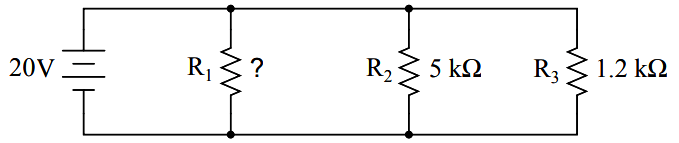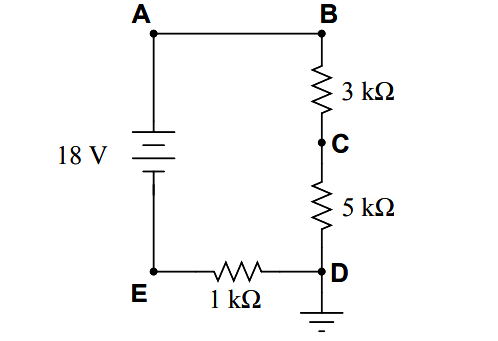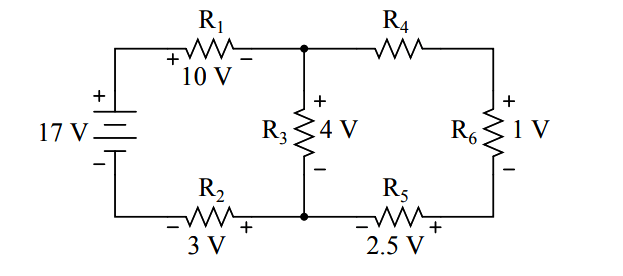# DC Circuits Multiple Choice Questions

DC Circuits Multiple Choice Questions

Question 1

Determine the voltage dropped across resistor R3 in this circuit:(A) 7.5 volts
(B) 3.0 volts
(C) 6.6 volts
(D) 15 volts
(E) 5.4 volts

Question 2

Calculate total current in this circuit:(A) 8.000 mA
(B) 7.835 mA
(C) 8.168 mA
(D) 493.8 µA
(E) 5.333 mA

Question 3

Calculate the necessary resistor value (R) to balance this bridge circuit:(A) 7.299 kΩ
(B) 6.464 kΩ
(C) 9.656 kΩ
(D) 8.501 kΩ
(E) 5.830 kΩ

Question 4

Calculate the necessary resistor size for R1 to make the total circuit current equal to 30 milliamps:(A) 2.143 kΩ
(B) 1.500 kΩ
(C) 967.7 Ω
(D) 666.7 Ω
(E) 310.0 Ω

Question 5

Calculate the current through resistor R4 in this circuit:(A) 1.226 mA
(B) 441.5 µA
(C) 1.668 mA
(D) 4.054 mA
(E) 8.994 mA

Question 6

Voltages and currents in an RC or LR time-constant circuit will settle to within 1% of their final value(s) in how many time constants?

(A) 10
(B) 3
(C) 5
(D) 2
(E) 4

Question 7

How much voltage will a DMM register if connected with the red test lead on point B and the black test lead on point D?(A) +15 volts
(B) -33 volts
(C) +33 volts
(D) -9 volts
(E) -15 volts

Question 8

Calculate the voltage between test point B and ground in this circuit:(A) 20 volts
(B) 18 volts
(C) 2 volts
(D) 6 volts
(E) 16 volts

Question 9

Determine the magnitude and direction of current through resistor R4 in this circuit:(A) 240 mA → (left to right)
(B) 160 mA ← (right to left)
(C) 200 mA → (left to right)
(D) 240 mA ← (right to left)
(E) 160 mA → (left to right)

Question 10

Determine the magnitude and polarity of the voltage across resistor R4 in this circuit:(A) – 1.0 volt + (negative on left, positive on right)
(B) – 7.0 volts + (negative on left, positive on right)
(C) + 0.5 volts – (positive on left, negative on right)
(D) – 3.5 volts + (negative on left, positive on right)
(E) + 4.0 volts – (positive on left, negative on right)